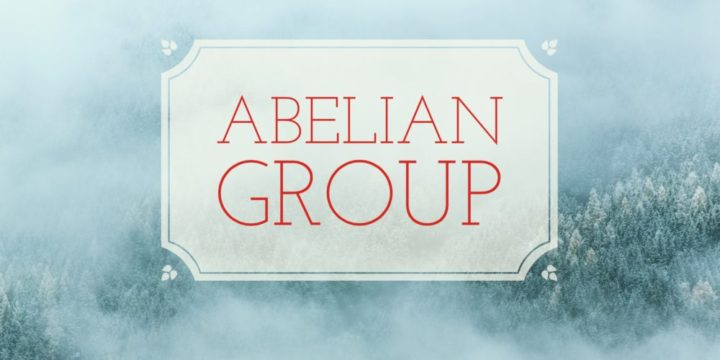# Two Quotients Groups are Abelian then Intersection Quotient is Abelian## Problem 148

Let $K, N$ be normal subgroups of a group $G$. Suppose that the quotient groups $G/K$ and $G/N$ are both abelian groups.

Then show that the group
$G/(K \cap N)$ is also an abelian group.Add to solve later

## Hint.

We use the following fact to prove the problem.

Lemma: For a subgroup $H$ of a group $G$, $H$ is normal in $G$ and $G/H$ is an abelian group if and only if the commutator subgroup $D(G)=[G,G]$ of $G$ is contained in $H$.

For a proof of this fact, see Commutator subgroup and abelian quotient group

## Proof.

By the lemma mentioned above, we know that $G/K$ is an abelian group if and only if the commutator subgroup $D(G)=[G,G]$ is contained in $K$.

Similarly, since $G/N$ is abelian, $D(G)$ is contained in $N$.
Therefore, the commutator subgroup $D(G) \subset K \cap N$. This implies, again by Lemma, that the quotient group
$G/(K \cap N)$ is an abelian group as required.Add to solve later

### 1 Response

1. 10/18/2016

[…] Next story Two quotients groups are abelian then intersection quotient is abelian […]

This site uses Akismet to reduce spam. Learn how your comment data is processed.

###### More in Group Theory##### Commutator Subgroup and Abelian Quotient Group

Let $G$ be a group and let $D(G)=[G,G]$ be the commutator subgroup of $G$. Let $N$ be a subgroup of...

Close